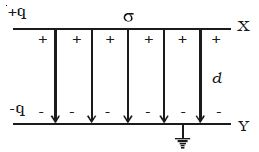Physics

# Describe Capacitance of a Parallel Plate Capacitor

The parallel plate capacitor consists of two parallel metal plates X and Y each of area A, separated by a distance d, having a surface charge density σ (figure). The medium between the plates is air. A charge +q is given to the plate X. It induces a charge –q on the upper surface of earthed plate Y. When the plates are very close to each other, the field is confined to the region between them. The electric lines of force starting from plate X and ending at the plate Y are parallel to each other and perpendicular to the plates.By the application of Gauss’s law, the electric field at a point between the two plates is,

E = σ/ε0

The potential difference between the plates X and Y is

V = 0d –E dr = 0d – σ/ε0 dr = σd/ε0

The capacitance (C) of the parallel plate capacitor

C = q/V = σA/ (σd/ε0) = ε0A/d

so, C = ε0A/d

The capacitance is directly proportional to the area (A) of the plates and inversely proportional to their distance of separation (d).